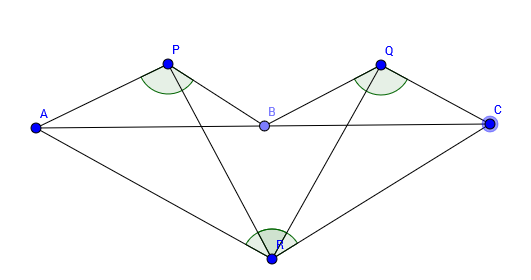# Don't Just Guess, Try To Prove!

Geometry Level 2Let $AC$ be a line segment in the plane and $B$ a point between $A$ and $C$. Construct an isosceles triangle $PAB$ and $QBC$ on one side of the segment $AC$ such that $\angle APB= \angle BQC=120^\circ$ and an isosceles triangle $RAC$ on the other side of $AC$ such that $\angle ARC=120^\circ$. Find the measure of $\angle PRQ$ in degrees.

×

Problem Loading...

Note Loading...

Set Loading...